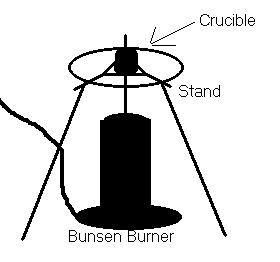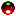CH - 120

Fall 2000

Water of Hydration

Experiment #2

Submitted by:

Andrew J. Buettner

Partner:

none

Report Due: March 6, 2003Objective

The objective of this lab is to determine the percent by weight of hydration in a compound. In order to accomplish this a compound with an unknown percent hydration is massed. Then the hydration is evaporated off. A new mass is taken. The difference in masses is used to calculate the percent by weight of hydration.Apparatus

A) Equipment used:

1) Bunsen burner

2) Metal stand

3) Crucible with lid

4) scale

5) Unknown substance #1

B) Diagram:Method

1) Obtain a clean crucible.

2) Mass the crucible.

3) Place about 1g of the unknown substance into the crucible.

4) Mass the crucible again.

5) Heat the crucible with the lid on it to prevent any substance from escaping but to allow the water vapor to escape.

6) Discontinue heating after ten minutes.

7) Allow the crucible to cool and mass the contents.

8) Heat again for an additional five minutes.

9) Allow the crucible to cool and mass the crucible with contents again.

10) Repeat steps 8 and 9 as needed until the mass remains relatively constant to ensure that the hydration has completely evaporated.Data

A) Mass of crucible and cover: 21.510g

B) Table of masses:

Initial

First heating

Final Heating

Mass

1.026g

.412g

.400g

C) Molecular mass of unknown #1: 142.2g/mol

D) Calculations:

1) Mass of water lost: .626g

2) Percentage of water in substance: 61.0%

3) Number of moles of water per mole of unknown 1: 12:1Answers to Lab Questions

Q: A solid hydrate weighing 2.125g was heated to drive off the water. The anhydrous remains weighed 1.044g. The substance has a formula weight of 104g/mole. What is the percent hydration and what is the ratio of moles water to moles substance?

A: 50.9% Water, 6 mole water:1 mole substanceConclusions

From this lab one could conclude that it is possible to obtain a percent hydration of a substance by only knowing the initial and final masses of the substance. Additionally it is proven that if the atomic weight of the substance is know then the moles of hydration per mole of substance ratio can be computer as well.Attachments

1. Original Handout

2. Data sheets

3. Calculations